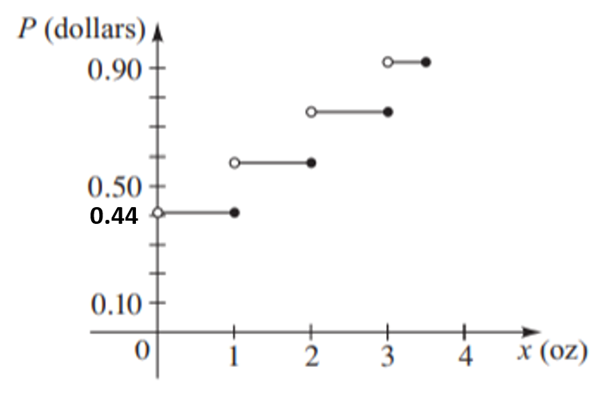# To Express : The postage P as a piecewise-defined function of the weight x of a letter with 0 &lt; x ≤ 3.5 and sketch the graph of the function.### Precalculus: Mathematics for Calcu...

6th Edition
Stewart + 5 others
Publisher: Cengage Learning
ISBN: 9780840068071### Precalculus: Mathematics for Calcu...

6th Edition
Stewart + 5 others
Publisher: Cengage Learning
ISBN: 9780840068071

#### Solutions

Chapter 2.2, Problem 83E
To determine

## To Express : The postage P as a piecewise-defined function of the weight x of a letter with 0<x≤3.5 and sketch the graph of the function.

Expert Solution

### Explanation of Solution

Given information :

The domestic postage rate for the first-class letters weighing 3.5 oz or less is 44 cents for the first ounce (or less) , plus 17 cents for each additional ounce (or a part of ounce).

Calculation :

It is known that 1 cent is equal to 0.01 dollars.

Let x be weight in ounce and P is measured in dollar

Compute the function P(x) for the interval 0 to 1 , that is P(x)=0.44 for the interval 0<x1

Compute the function P(x) for the interval 1 to 2 , therefore,

P(x)=0.44+0.17=0.61

Therefore, the function is written as, P(x)=0.61 if 1<x2

Compute the function P(x) for the interval 2 to 3 ,

P(x)=0.61+0.17=0.78

Therefore, the function is written as P(x)=0.78 if 2<x3

Compute the function P(x) for the interval 3 to 3.5 ,

P(x)=0.78+0.17=0.95

Therefore, the function is written as, P(x)=0.95 if 3<x3.5

Hence, the function is represented as P(x)={0.44 if 0<x10.61 if 1<x20.78 if 2<x30.95 if 3<x3.5

Graph:

The graph is thus obtained as:### Have a homework question?

Subscribe to bartleby learn! Ask subject matter experts 30 homework questions each month. Plus, you’ll have access to millions of step-by-step textbook answers!